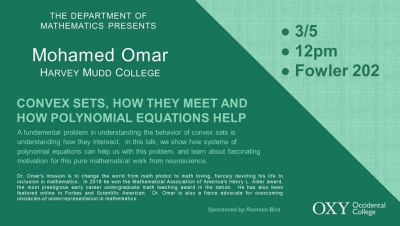# Talk by Dr. Mohamed Omar: Convex Sets, How They Meet and How Polynomial Equations Help

Join the Department of Mathematics of a talk by Dr. Mohamed Omar of Harvey Mudd College on "Convex Sets, How They Meet and How Polynomial Equations Help"!

Mar5
12:00 pm
2020-03-05 12:00:00 2020-03-05 12:00:00 Talk by Dr. Mohamed Omar: Convex Sets, How They Meet and How Polynomial Equations Help

A fundamental problem in understanding the behavior of convex sets is understanding how they intersect. In this talk, we show how systems of polynomial equations can help us with this problem, and learn about the fascinating motivation of this pure mathematical work from neuroscience.

America/Los_Angeles public
Location:
Event Date: Thursday, March 5, 2020

A fundamental problem in understanding the behavior of convex sets is understanding how they intersect. In this talk, we show how systems of polynomial equations can help us with this problem, and learn about the fascinating motivation of this pure mathematical work from neuroscience.